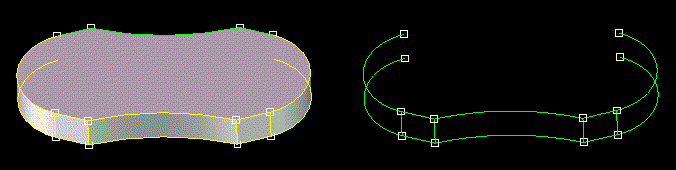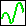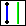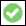> > Convert existing geometry into a 3D curve

Convert existing geometry into a 3D curve
The 3D Curve Convert function provides an indirect method to create 3D spline curves from existing geometry. By selecting a series of arbitrary edges on an existing part, the Convert function will generate spline curve approximations of that geometry. You can then modify the spline curves, if desired, using the Modify Spline functions.
The figure shows how selected edges of a solid part can be converted into 3D spline curves and then combined in the wire part shown on the right.To create a 3D curve by converting existing geometry,
1. Click 3D Geometry and then, in the 3D Curve group, click More next toSpline 3D.
2. ClickConvert Curve in the Indirect section. The Convert dialog box opens.
3. Type a name for Part.
4. Click Edges and choose the edges with the Select tool.
5. Clickto complete the operation.
To display only the wire part, either delete the source geometry, or deactivate the part in the Structure Browser.